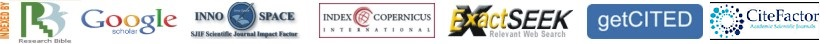### Implementation of Re configurable FIR Filter Using Distributed Arithmetic in FPGA

Utpal Kumar Paul, Deepanwita Sar, Depanwita Debnath

#### Abstract

Finite impulse response (FIR) filters have the advantage of linear phase, guaranteed stability, fewer finite precision errors, and efficient implementation. In contrast, they have a major disadvantage of higher order need (more coefficients) than IIR counterpart with comparable performance. The higher order demand imposes more hardware requirements, arithmetic operations, area usage, and power consumption when designing and fabricating the filter. This paper presents an algorithm proposed for modifying values and the number of non-zero coefficients used to represent the FIR digital pulse shaping filter response and it provide to reconfigure the parameters of the filter like numbers of bits in both inputs and outputs and the number of coefficients by which we can tune the resolution of the filter. With this algorithm, the FIR filter frequency and phase response can be represented with a minimum number of non-zero coefficients. Therefore, reducing the arithmetic complexity needed to get the filter output. Consequently, the system characteristic i.e. power consumption, area usage, and processing time are also reduced. Here the DA usage eliminates the need for multipliers when implementing the multiply and accumulate unit (MAC) and the proposed algorithm will reduce the number of adders and addition operations needed through the minimization of the non-zero values coefficients to get the filter output.

PDF

### Refbacks

• There are currently no refbacks.